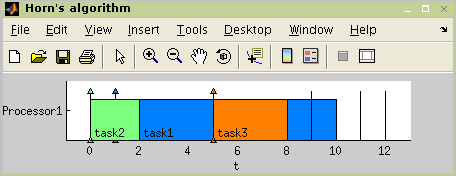"; } ?> Quick Start

# TORSCHE Scheduling Toolbox for Matlab

## TORSCHE – Quick Start

### 1. Software Requirements

TORSCHE Scheduling Toolbox for Matlab (0.4.0) currently supports MATLAB 6.5 (R13) and higher versions. If you want to use the toolbox on different platforms than MS-Windows or Linux on PC (32bit) compatible, some algorithms must be compiled by a C/C++ compiler. We recommend to use Microsoft Visual C/C++ 7.0 and higher under Windows or gcc under Linux.

### 2. Installation

Download the toolbox from web and unpack Scheduling toolbox into the directory where Matlab toolboxes are installed (most often in `<Matlab root>\toolbox` on Windows systems and on Linux systems in `<Matlab root>/toolbox`). Run Matlab and add two new paths into directories with Scheduling toolbox and demos, e.g.:

```>> addpath(path,'c:\matlab\toolbox\scheduling')

Several algorithms in the toolbox are implemented as Matlab MEX-files (compiled C/C++ files). Compiled MEX-files for MS-Windows and Linux on PC (32bit) compatible are part of this distribution. If you use the toolbox on a different platform, please compile these algorithms using command `make` from `\scheduling` directory (in Matlab environment). Before that, please specify the compiler using command `mex -setup` from (also in Matlab environment). We suggest to use Microsoft Visual C/C++ or gcc compilers.

### 3. Help

To display a list of all available commands and functions please type

`>> help scheduling`

To get help on any of the toolbox commands (e.g. `task`) type

`>> help task`

To get help on overloaded commands, i.e. commands that do exist somewhere in Matlab path (e.g. `plot`) type

`>> help task/plot`

Or alternatively type `help plot` and then select `task/plot` at the bottom line line of the help text.

### 4. How to Solve Your Scheduling Problems

Solving procedure of your scheduling problem can be divided into four basic steps:

1. Define a set of tasks.

2. Define the scheduling problem.

3. Run the scheduling algorithm.

Task is defined by command `task`, for example:

```>> t1 = task('task1', 5, 1, inf, 12)
Processing time: 5
Release time:    1
Due date:        12```

This command defines task with name ``task1", processing time 5, release time 1, and duedate at time 12. In the same way we can define next tasks:

```>> t2 = task('task2', 2, 0, inf, 11);

To create a set of tasks use command `taskset`:

```>> T = taskset([t1 t2 t3])

For short:

```>> T = [t1 t2 t3]

Due to great variety of scheduling problems, it is not easy to choose a proper algorithm. For easier selection of the proper algorithm, the toolbox uses a notation, proposed by [Graham79] and [Błażewicz83], to classify scheduling problems. Those classifications are created by command `problem`:

```>> p=problem('1|pmtn,rj|Lmax')
1|pmtn,rj|Lmax```

Now we can execute the scheduling algorithm, for example Horn's algorithm:

```>> TS = horn(T,p)
There is schedule: Horn's algorithm
Solving time: 0.29s```

The final schedule, given by Gantt chart ,is shown in Figure 2.1, “The taskset schedule”. The figure is plotted by:

`>> plot(TS)`Data from the Matlab workspace can be saved and loaded by standard commands `save` and `load`. For example:
```>> save file1